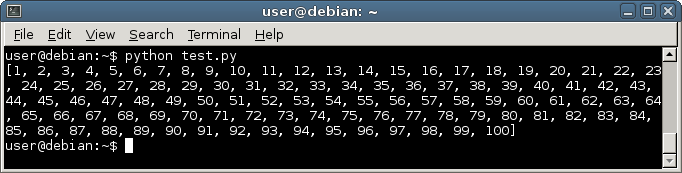The range() function generates a list of numbers. This is very useful when creating new lists or when using for loops: it can be used for both.

In practice you rarely define lists yourself, you either get them from a database, the web or generate them using range().

Related course: Complete Python Programming Course & Exercises

## Python range() parameters

The range() function takes parameter, which must be integers. They can be both positive and negative.
By default, it creates a list of numbers starting from zero, as parameter the stop value is defined

But you can define the starting number of the sequence and then step size.

## Python’s range() example

Lets say you want to create a list of 100 numbers. To do that, you can use the range() function. By calling `list(range(100))` it returns a list of 100 numbers. Writing them out by hand would be very time consuming, so instead use the range function:

Python starts counting from zero. Now what if you want to count from 1 to 100?A third parameter defines the step size, by default its one. Range can be used in a for loop:

Some other examples which have a step size parameter:

If you are a beginner, then I highly recommend this book.

## Exercise

Try the exercises below

1. Create a list of one thousand numbers
2. Get the largest and smallest number from that list
3. Create two lists, an even and odd one.